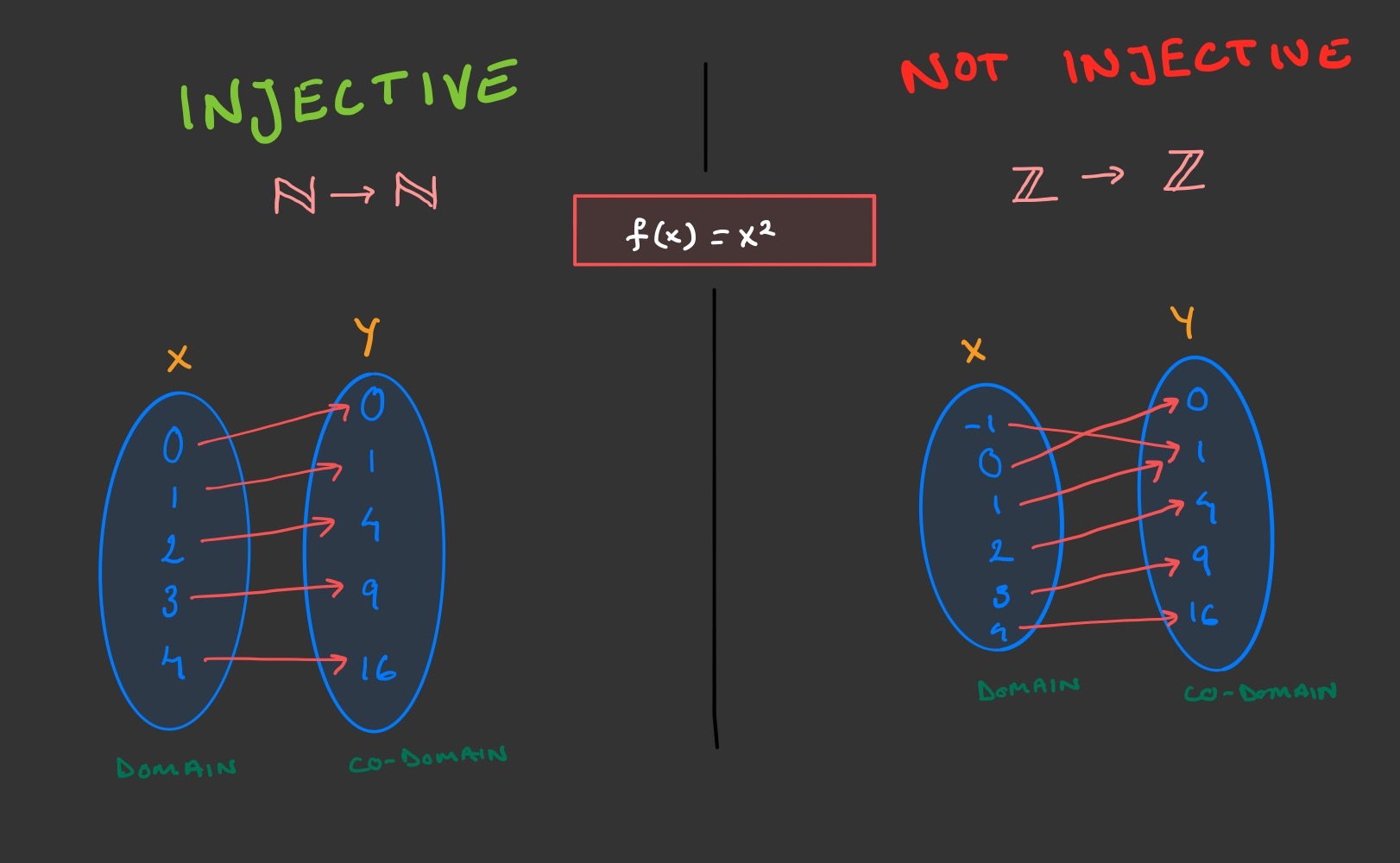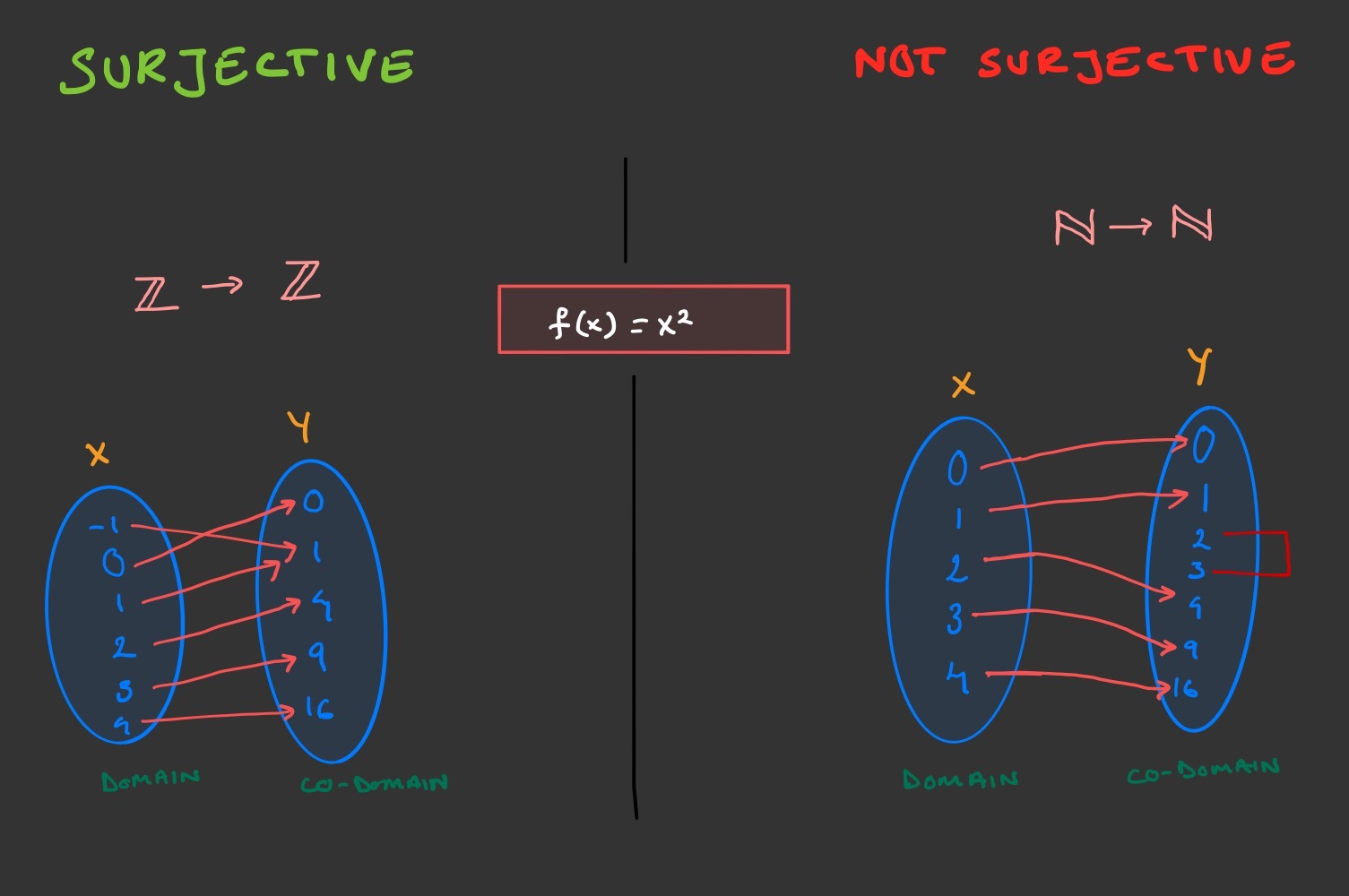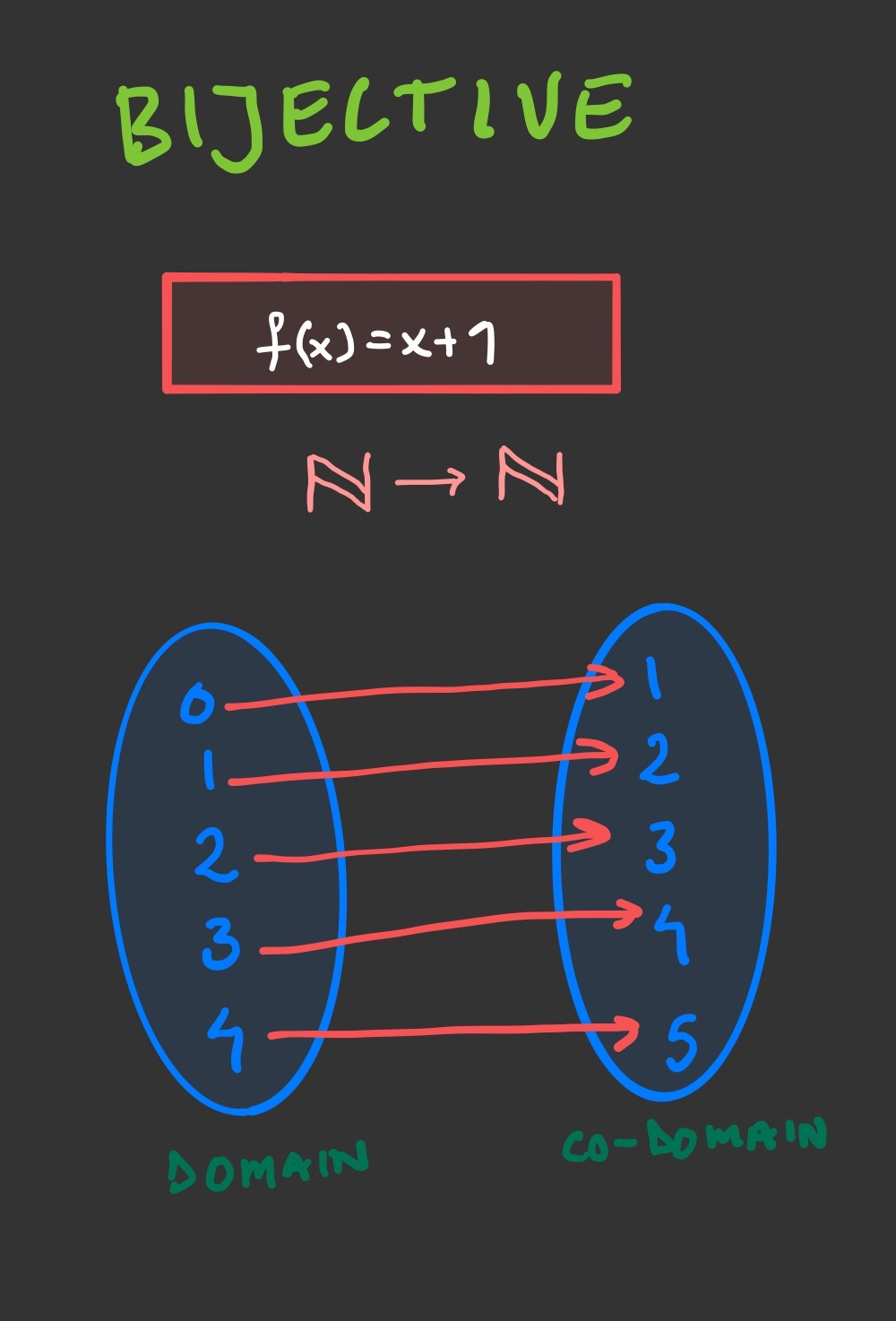# Functions

First, let’s define some things.

Set: A collection of unique objects, these can be anything (shapes, letters, numbers, etc) but in this case we’ll be referring to a collection of unique numbers.

Domain: A set of inputs for a function.

Co-domain: A set of outputs for a function.

$$\Bbb{N}$$ (natural numbers): a set of positive whole numbers, including 0

$$\Bbb{Z}$$ (integers): a set of positive and negative whole numbers, including 0.

According to Wikipedia, a function is “a binary relation over two sets that associates to every element of the first set exactly one element of the second set”. When we speak about functions, we’re talking about how we map a domain to a co-domain, what instructions do we need to give to a domain to ensure that it’s mapped to the correct item in the co-domain. If we had a domain of { 1, 2, 3, 4} and a co-domain of {2, 4, 6, 8}, we can figure out that the instruction we’d need to give the domain is multiply each thing by 2. So the function would look like $$f(x) = x * 2$$. Not every given instruction is a function though, what makes a function a function is that every input must produce exactly one output. So the function $$f(x) = \pm\sqrt x$$ is not a function because $$f(9)$$ can equal both 3 and -3.## Injective Functions

These are also known as one-to-one functions, and mean that every input has at most one output, and every output has at most one input. Another way to think of it is, every item in the co-domain can have up to one output, which means an item may have no output. E.g.

If we have a set of natural numbers $$\Bbb{N}$$ (the domain) that maps onto another set of $$\Bbb{N}$$ (the co-domain), the function $$f(x) = x^2$$ is injective because for every inputted natural number, there is an outputted natural number. However, if we change those sets to include negative numbers so instead map integers to integers, $$\Bbb{Z} → \Bbb{Z}$$, then the same function is not injective because $$f(1) = 1$$ and $$f(-1) = 1$$. When we use integers, we have two items in the domain that map to an item in the codomain.## Surjective Functions

Also known as on-to functions, are functions where the input has one output and every output has at least one input, which means an output can have more than one input. If we use our previous example $$f(x) = x^2$$ if $$\Bbb{Z} → \Bbb{Z}$$ then the function is surjective, since, as we saw before, both $$f(1)$$ and $$f(-1)$$ equal 1. However, if we apply the same function to only sets of $$\Bbb{N}$$ like we did before, then the function stops being surjective since there isn’t an input where $$f(x) = 2$$ or where $$f(x) = 3$$, meaning that there will be some output that have 0 (or less than one) input.## Bijective Functions

These are functions that are both injective and surjective, so every input must map to exactly one output and every output must map to exactly one input. If we have two sets, $$\Bbb{N} → \Bbb{N}$$, then the function $$f(x) = x + 1$$ would be bijective since there can be no input which doesn’t have exactly one output.## Why does this matter?

The concept of a function exists in programming and is a programming style, Functional Programming. Stanford’s CS103 course has a slide deck which goes into a lot of detail about the similarities but essentially, you can think of programming functions in a similar way as math functions. They take an input from a domain, apply an instruction and return an exactly one output within a co-domain, and an input should always return the same output.

If you worked with relational databases, you may also see similarities in how a database references relationships between data and how functions reference relationships between data. An injective function is similar to a 1-many relationship, a surjective function is similar to a many-1 relationship and a bijective function is similar to a 1-1 relationship.